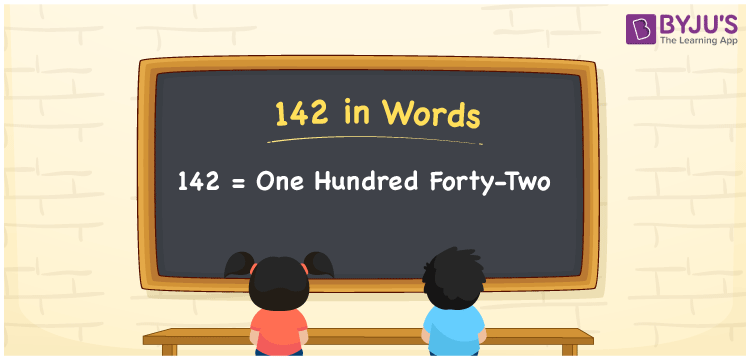# 142 in Words

142 in words is written as One hundred forty-two. In both the International System of Numerals and the Indian System of Numerals, 142 is written as One hundred forty-two. The number 142 is a Cardinal Number as it represents some quantity. For example, “there are 142 chairs in this room”.

 142 in Words One hundred forty-two One hundred forty-two in Number 142

## 142 in English Words

We write 142 in English Words using the letters of the English alphabet. Therefore, we read 142 in English as “One hundred forty-two.”## How to Write 142 in Words?

To write 142 in words, we shall use the place value chart. In the place value chart, write 1 in the hundreds, 4 in the tens, and 2 in the ones, respectively. Now let us make a place value chart to write the number 142 in words.

 Hundreds Tens Ones 1 4 2

Thus, we can write the expanded form as

1 × Hundred + 4 × Ten + 2 × One

= 1 × 100 + 4 × 10 + 2 × 1

= 100 + 40 + 2

= 142

= One hundred forty-two.

142 is a natural number, the successor of 141 and the predecessor of 143.

142 in words – One hundred forty-two

• Is 142 an odd number? – No
• Is 142 an even number? – Yes
• Is 142 a perfect square number? – No
• Is 142 a perfect cube number? – No
• Is 142 a prime number? – No
• Is 142 a composite number? – Yes

## Frequently Asked Questions on 142 in Words

Q1

### How to write 142 in words?

142 in words is written as One hundred forty-two.
Q2

### How to write 142 in the International and Indian System of Numerals?

In both, the system of numerals, 142 in words, is written as One hundred forty-two.
Q3

### What are the factors of 142?

The factors of 142 are 1, 2, 71 and 142.